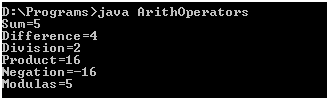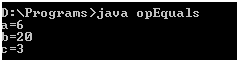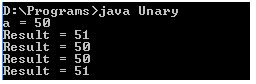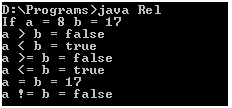# Java Operators and Expressions

To perform any kind of evaluation Java Operators and Expressions are required in a program. An expression is any statement that produces a value. Most of the expressions are mathematical such as:

int i=10, y=5;

int z = x * y;

The last statement in this example is an expression. This example expression multiplies the two values stored in variables x and y and stores the result in z. The result produced by an expression is called a return value.

Most expressions in Java make use of operators. Operators are symbols that perform some arithmetic and logical operations on its operands and produces meaningful results. Operands are the data values that are involved in the operations. The operands of the expression are always evaluated from left to right. An operator requires one or more operands to perform any operation.  There are various operators that can be used in Java. These are discussed below.

## Arithmetic Operators

The operators which are used to perform basic mathematical calculations in our program are known as arithmetic operators. These are:

 Operator Meaning + – * / % ++ — += -= *= /= Addition Subtraction Multiplication Division Modulus Increment Decrement Addition assignment Subtraction assignment Multiplication assignment Division assignment

You will get a better understanding of these with the help of the following examples.

### Basic Arithmetic Operators

These are used for performing mathematical operations.

// Example of basic arithmetic operators

``` class ArithOperators
{
public static void main(String args [])
{
int a = 2 + 3;

int b = 6 - 2;

int c = b / 2;
int d = c * 8;
int e = -d;
int f = a % 10;
System.out.println("Sum=" + a);
System.out.println("Difference=" + b);
System.out.println("Division=" + c);
System.out.println("Product=" + d);
System.out.println("Negation=" + e);
System.out.println("Modulas=" + f);
}
}```

Output:### Arithmetic Assignment Operators

These are used to combine an arithmetic operator with an assignment operator.

// Example illustrating arithmetic assignment operators

``` class opEquals
{
public static void main(String args [])
{
int a = 1;
int b = 2;
int c = 3;
a += 5;
b *= 10;
c += a * b;
c %= 5;
System.out.println("a=" + a);
System.out.println("b=" + b);
System.out.println("c=" + c);
}
}```

### Output:### Increment and Decrement Operators

These are used to increase or decrease the value of an operator by 1. The statement x++ is the same as x = x + 1.

// Example illustrating increment and decrement operators

``` class Unary
{
public static void main(String args [])
{
int a = 50, result;
System.out.println("a = " + a);
result = ++a;
System.out.println("Result = " + result);
result = --a;
System.out.println("Result = " + result);
result = a++;
System.out.println("Result = " + result);
result = a--;
System.out.println("Result = " + result);
}
}```

Output:NOTE: – The difference in y = x++ and y = ++x is that in x++ the value of x is first assigned to the variable y and then x is incremented, whereas, in ++x the value of x is first incremented and then assigned to the variable y.

## Relational Operators

These operators determine the relationship between both the operands. The relational operators are as follows:

 Operator Symbol Format Greater than Less than Greater than or equal to Less than or equal to Equal to Not equal to > < >= <= = != a > b a < b a >= b a <= b a = b a != b

For example:

``` class Rel
{
public static void main(String args [])
{
int a = 8, b1=17;
System.out.println("If a = " + a + " b = " + b1);
System.out.println("a > b = " + (a > b1));
System.out.println("a < b = " + (a < b1));
System.out.println("a >= b = " + (a >= b1));
System.out.println("a <= b = " + (a <= b1));
System.out.println("a = b = " + (a = b1));
System.out.println("a != b = " + (a != b1));
}
}```

Output:## Logical Operators

These operators are used to construct complex decision making expressions. They provide the result as true or false depending on the expression. These operators are as follows:

 Operator Meaning Result && || ! Logical and Logical or Logical not It returns true if both the expressions are evaluated to true. It returns true if either expression evaluates to true. If expression returns true then not returns false and vice versa

## Assignment Operator

The = symbol works as an assignment operator. Its general form is

var = expression;

For example: x = y = z = 100;